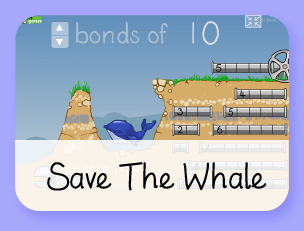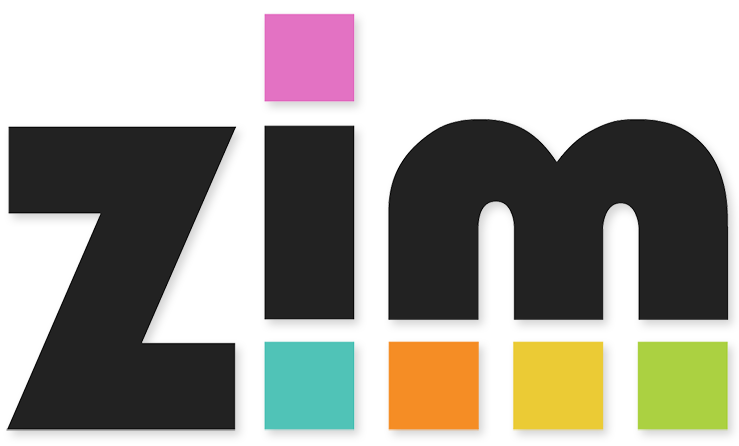# Part-Part-Whole

Learning Objectives:
• Y1: To represent and use number bonds and related subtraction facts within 20
• Y1: To add and subtract one-digit and two-digit numbers to 20, including zero
• Y1: To solve one-step problems that involve addition and subtraction, using concrete objects and pictorial representations, and missing number problems such as 7 = missing number – 9.
• Y2: To solve problems with addition and subtraction using concrete objects and pictorial representations, including those involving numbers, quantities (and measures)
• Y2: To recall and use addition and subtraction facts to 20 fluently, and derive and use related facts up to 100
• Y2: To add and subtract numbers using concrete objects, pictorial representations, and mentally, including... a two-digit number and ones, a two-digit number and tens, two two-digit numbers, adding three one-digit numbers
• Y2: To show that addition of two numbers can be done in any order (commutative) and subtraction of one number from another cannot
• Y2: To recognise and use the inverse relationship between addition and subtraction and use this to check calculations and solve missing number problems
This is a demonstration tool suitable when using the 'Part-Part-Whole Model' for addition and subtraction.

Choose which manipulatives you wish to use. To change your manipulatives at any time you can press the 'change' button.

You can add text by usng the keypad and then dragging it on.

You can remove anything by dragging it to the right-hand side.

Have fun!

Build on the learning with
these games:Made using theandlibraries.